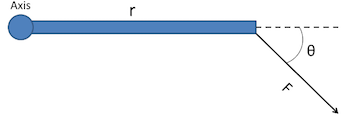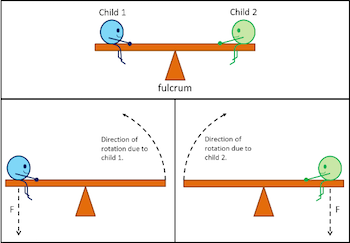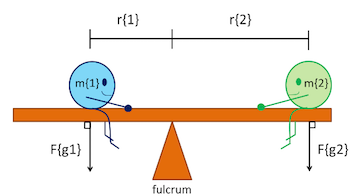# What is Torque? - Definition, Equation & Calculation

An error occurred trying to load this video.

Try refreshing the page, or contact customer support.

Coming up next: Friction: Definition and Types

### You're on a roll. Keep up the good work!

Replay
Your next lesson will play in 10 seconds
• 0:00 Torque in Everyday Life
• 0:50 Physics of Torque
• 2:10 Rotational Equilibrium
• 3:33 Seesaw Stalemate Problem
• 6:11 Lesson Summary

Want to watch this again later?

Timeline
Autoplay
Autoplay
Speed

#### Recommended Lessons and Courses for You

Lesson Transcript
Instructor: Damien Howard

Damien has a master's degree in physics and has taught physics lab to college students.

You've probably heard of torque before, maybe while discussing cars. Now learn what it really is, and what it has to do with rotational equilibrium. Then, work through an example problem that combines the two.

## Torque in Everyday Life

When we hear the term 'torque' brought up, it's most often in the context of automobiles. Torque is one of the terms commonly thrown around to describe how powerful a car is, but what exactly does it mean? In a car, torque is the force that pistons put on the crankshaft, causing it and the wheels to turn.

While often considered an automotive term, torque is actually a general physics term that has many applications. Torque is defined as a twisting force that tends to cause rotation. We call the point where the object rotates the axis of rotation. You use torque every day without realizing it. You apply torque three times when you simply open a locked door. Turning the key, turning the doorknob, and pushing the door open so it swings on its hinges are all methods of applying a torque.

## Physics of Torque

In order to find a linear force we need to know a mass and an acceleration. However, torque is a little different, thanks to rotation being involved. Think about opening a door. Where do you push on it when you want it to open? You push on the side of the door where there are no hinges because pushing on the side with the hinges would make it much harder to open. So for torque, we need to know not only the mass and acceleration of a linear force, but also how far that force is from the axis of rotation, since we can get different results depending on that, as well. We can see this in the diagram and equation for torque.T = F * r * sin(theta)

T = torque

F = linear force

r = distance measured from the axis of rotation to where the linear force is applied

theta = the angle between F and r

In our equation, sin(theta) has no units, r has units of meters (m), and F has units of Newtons (N). Combining these together, we see that a unit of torque is a Newton-meter (Nm).

Finally, theta is needed to take into account the direction from which the linear force is being applied. The force will not always be pushed from straight on like a door. It can come from many different angles.

## Rotational Equilibrium

So, we've seen how one torque can work on an object, but you can easily have more than one torque being applied at once. Think back to the car engine. In every car, there is more than one piston applying torque to the crankshaft. In this case there is a total torque that is the sum of each individual torque.

Total T = T{1} + T{2} + ... + T{n}

In this equation, n is the total number of torques being applied to the object. There is also a special case of this called rotational equilibrium. This is where the addition of all the torques acting on an object equals zero. When this happens, this can mean that there is no torque acting on the object, or all the torques acting on the object are canceling each other out. In order to visualize torques canceling out, let's look at a simple case with two torques: a seesaw.In the top part of the image, two children are sitting on a seesaw that isn't moving. They are balanced at the axis of rotation, which is the fulcrum in the case of a seesaw. Both children are exerting a force down with their weight, otherwise known as the force due to gravity. Child 1 is trying to rotate the seesaw counterclockwise, and child 2 is trying to rotate it clockwise. As long as the magnitudes of the two torques are the same, they cancel each other out since they are trying to move the seesaw in opposite directions.

## Seesaw Stalemate Problem

Let's look at an example calculation using both rotational equilibrium and the equation for torque.The seesaw in the image is in rotational equilibrium and not moving. We want to find how far child 2 to the right is from the axis of rotation at the fulcrum. Child 1 to the left has a mass of 38 kg and is 4 m away from the fulcrum. Child 2 has a mass of 25 kg.

To unlock this lesson you must be a Study.com Member.

### Register to view this lesson

Are you a student or a teacher?

### Unlock Your Education

#### See for yourself why 30 million people use Study.com

##### Become a Study.com member and start learning now.
Back
What teachers are saying about Study.com

### Earning College Credit

Did you know… We have over 160 college courses that prepare you to earn credit by exam that is accepted by over 1,500 colleges and universities. You can test out of the first two years of college and save thousands off your degree. Anyone can earn credit-by-exam regardless of age or education level.